Related Articles

# AI Driven Snake Game using Deep Q Learning

• Difficulty Level : Easy
• Last Updated : 18 Jul, 2021

Introduction: This Project is based on Reinforcement Learning which trains the snake to eat the food present in the environment.

A sample gif is given below, that you can get an idea of what we are going to build.

Attention geek! Strengthen your foundations with the Python Programming Foundation Course and learn the basics.

To begin with, your interview preparations Enhance your Data Structures concepts with the Python DS Course. And to begin with your Machine Learning Journey, join the Machine Learning - Basic Level Course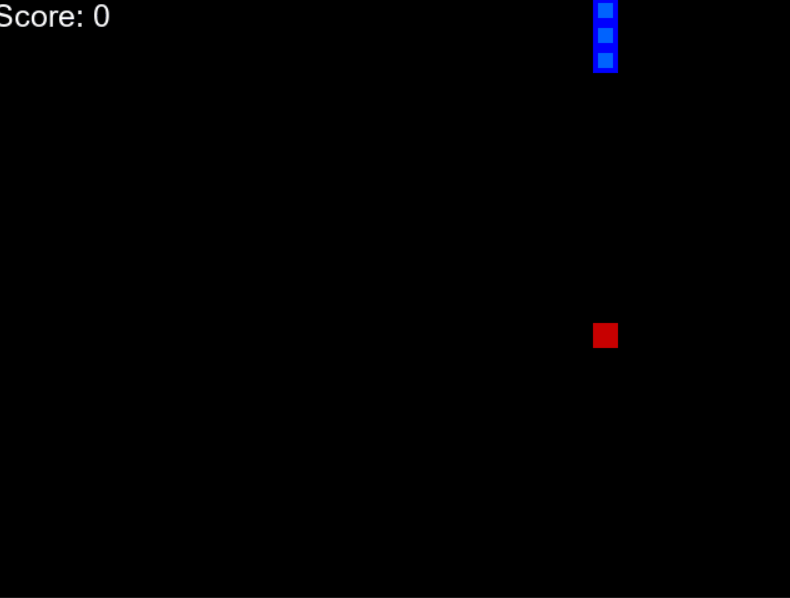Snake Driven by AI

The Prerequisite for this project are:

• Reinforcement Learning
• Deep Learning (Dense Neural Network)
• Pygame

To understand how can we manually build this snake 2D animation simulation using pygame, please follow the link: https://www.geeksforgeeks.org/snake-game-in-python-using-pygame-module/

After building the basic snake game now we will focus on how to apply Reinforcement learning to it.

We have to create three Modules in this project:

1. The Environment (the game that we just build)
2. The Model (Reinforcement model for move prediction)
3. The Agent (Intermediary between Environment and Model)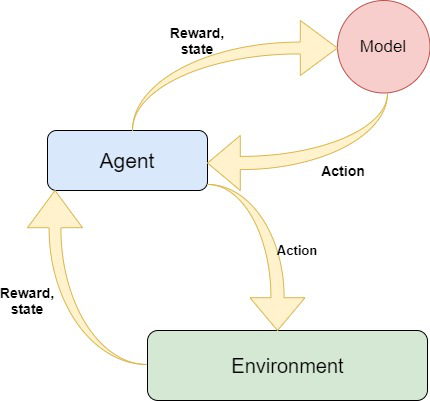### Algorithm:

We have snake and food on the board randomly placed.

• Calculate the state of the snake using the 11 values. if any the condition is true then set that value to zero else set one.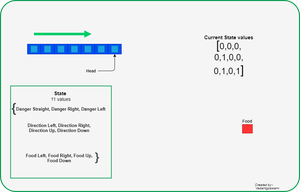How 11 states are defined

Based on the current Head position agent will calculate the 11 state values as described above.

• After getting these state, agent would pass this to the model and get the next move to perform.
• After executing the next state calculate the reward. Rewards are defined as below:
• Eat food : +10
• Game Over : -10
• Else : 0
•   Update the Q value (will be discussed later) and Train the Model.
•   After analyzing the algorithm now we have to build the idea to proceed for coding this algorithm.

### The Model: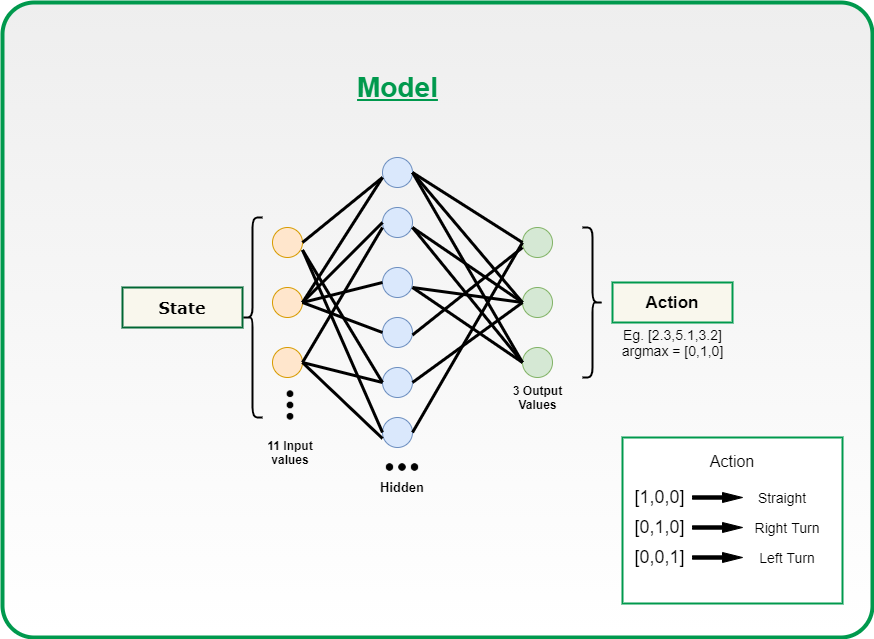Neural network Model

The model is designed using Pytorch, but you can also use TensorFlow based on your comfort.

We are using Dense neural network with an input layer of size 11 and one dense layer with 256 neurons and output of 3 neurons. You can tweak these hyper parameters to get the best result.

### How models works ?

• The game starts, and the Q-value is randomly initialized.
• The system gets the current state s.
• Based on s, it executes an action, randomly or based on its neural network. During the first phase of the training, the system often chooses random actions to maximize exploration. Later on, the system relies more and more on its neural network.
• When the AI chooses and performs the action, the environment gives a reward. Then, the agent reaches the new state and it updates its Q-value according to the Bellman equation. This equation you had definitely covered in the reinforcement learning course. If not you can refer Q-learning Mathematics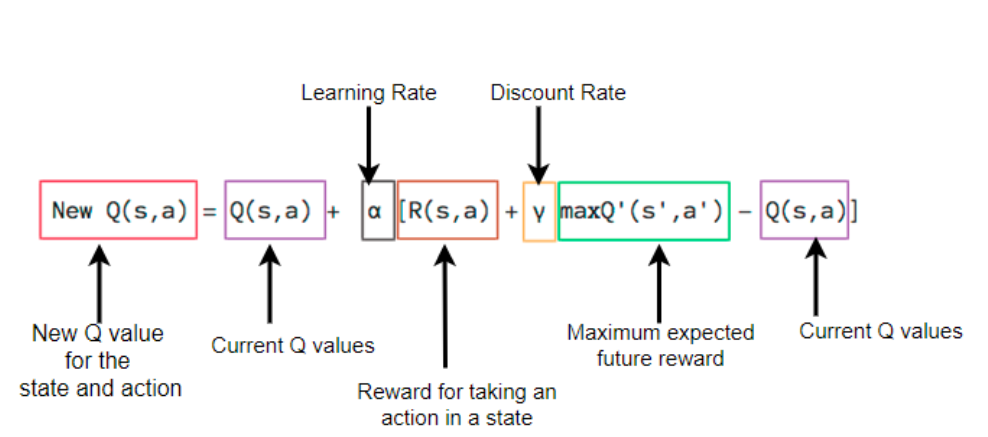Bellman Equation

• Also, for each move, it stores the original state, the action, the state reached after performed that action, the reward obtained and whether the game ended or not. This data is later sampled to train the neural network. This operation is called Replay Memory.
• These last two operations are repeated until a certain condition is met (example: the game ends).

The heart of this project is the model that you are going to train because the correctness of the move that the snake would play will all depend on the quality of the model you had built. So I would like to explain you this using the code in parts.

Part-I

```1. Creating a class named Linear_Qnet for initializing the linear neural network.
2. The function forward is used to take the input(11 state vector) and pass it through the
Neural network and apply relu activation function and give the output back i.e the next
move of 1 x 3 vector size. In short, this is the prediction function that would be called by the agent.
3. The save function is used to save the trained model for future use.```

## Python3

 `class` `Linear_QNet(nn.Module):``    ``def` `__init__(``self``, input_size, hidden_size, output_size):``        ``super``().__init__()``        ``self``.linear1 ``=` `nn.Linear(input_size, hidden_size)``        ``self``.linear2 ``=` `nn.Linear(hidden_size, output_size)`` ` `    ``def` `forward(``self``, x):``        ``x ``=` `F.relu(``self``.linear1(x))``        ``x ``=` `self``.linear2(x)``        ``return` `x`` ` `    ``def` `save(``self``, file_name``=``'model_name.pth'``):``        ``model_folder_path ``=` `'Path'``        ``file_name ``=` `os.path.join(model_folder_path, file_name)``        ``torch.save(``self``.state_dict(), file_name)`

Part-II

```1. Initialising QTrainer class
∗ setting the learning rate for the optimizer.
* Gamma value that is the discount rate used in Bellman equation.
* initialising the Adam optimizer for updation of weight and biases.
* criterion is the Mean squared loss function.
2. Train_step function
* As you know that PyTorch work only on tensors, so we are converting all the input
to tensors.
* As discussed above we had a short memory training then we would only pass one value
of state, action, reward, move so we need to convert them into a vector, so we had used
unsqueezed function .
* Get the state from the model and calculate the new Q value using the below formula:
Q_new = reward + gamma * max(next_predicted Qvalue)
* calculate the mean squared error between the new Q value and previous Q value and
backpropogate that loss for weight updation. ```

## C++

 `class` `QTrainer:``    ``def __init__(self,model,lr,gamma):``#Learning Rate for Optimizer``        ``self.lr = lr``#Discount Rate``        ``self.gamma = gamma``#Linear NN defined above.``        ``self.model = model``#optimizer for weight and biases updation``        ``self.optimer = optim.Adam(model.parameters(),lr = self.lr)``#Mean Squared error loss function``        ``self.criterion = nn.MSELoss()``         ` ` ` `     ` `    ``def train_step(self,state,action,reward,next_state,done):``        ``state = torch.tensor(state,dtype=torch.``float``) ``        ``next_state = torch.tensor(next_state,dtype=torch.``float``)``        ``action = torch.tensor(action,dtype=torch.``long``) ``        ``reward = torch.tensor(reward,dtype=torch.``float``)`` ` `#only one parameter to train,                              \``    ``Hence convert to tuple of shape(1, x)``        ``if``(len(state.shape) == 1):``#(1, x)``            ``state = torch.unsqueeze(state,0) ``            ``next_state = torch.unsqueeze(next_state,0) ``            ``action = torch.unsqueeze(action,0) ``            ``reward = torch.unsqueeze(reward,0) ``            ``done = (done, )`` ` `# 1. Predicted Q value with current state``        ``pred = self.model(state)``        ``target = pred.clone()``        ``for` `idx in range(len(done)):``            ``Q_new = reward[idx]``            ``if` `not done[idx]:``                ``Q_new = reward[idx] + ``                  ``self.gamma * torch.max(self.model(next_state[idx])) ``            ``target[idx][torch.argmax(action).item()] = Q_new``# 2. Q_new = reward + gamma * max(next_predicted Qvalue)``#pred.clone()``#preds[argmax(action)] = Q_new``        ``self.optimer.zero_grad()``        ``loss = self.criterion(target,pred)``        ``loss.backward() # backward propogation of loss`` ` `        ``self.optimer.step()`

### The Agent

• Get the current state of the snake from the environment.

## Python

 `def` `get_state(``self``, game):``    ``head ``=` `game.snake[``0``]``    ``point_l ``=` `Point(head.x ``-` `BLOCK_SIZE, head.y)``    ``point_r ``=` `Point(head.x ``+` `BLOCK_SIZE, head.y)``    ``point_u ``=` `Point(head.x, head.y ``-` `BLOCK_SIZE)``    ``point_d ``=` `Point(head.x, head.y ``+` `BLOCK_SIZE)`` ` `    ``dir_l ``=` `game.direction ``=``=` `Direction.LEFT``    ``dir_r ``=` `game.direction ``=``=` `Direction.RIGHT``    ``dir_u ``=` `game.direction ``=``=` `Direction.UP``    ``dir_d ``=` `game.direction ``=``=` `Direction.DOWN`` ` `    ``state ``=` `[``        ``# Danger Straight``        ``(dir_u ``and` `game.is_collision(point_u))``or``        ``(dir_d ``and` `game.is_collision(point_d))``or``        ``(dir_l ``and` `game.is_collision(point_l))``or``        ``(dir_r ``and` `game.is_collision(point_r)),`` ` `        ``# Danger right``        ``(dir_u ``and` `game.is_collision(point_r))``or``        ``(dir_d ``and` `game.is_collision(point_l))``or``        ``(dir_u ``and` `game.is_collision(point_u))``or``        ``(dir_d ``and` `game.is_collision(point_d)),`` ` `        ``# Danger Left``        ``(dir_u ``and` `game.is_collision(point_r))``or``        ``(dir_d ``and` `game.is_collision(point_l))``or``        ``(dir_r ``and` `game.is_collision(point_u))``or``        ``(dir_l ``and` `game.is_collision(point_d)),`` ` `        ``# Move Direction``        ``dir_l,``        ``dir_r,``        ``dir_u,``        ``dir_d,`` ` `        ``# Food Location``        ``game.food.x < game.head.x,  ``# food is in left``        ``game.food.x > game.head.x,  ``# food is in right``        ``game.food.y < game.head.y,  ``# food is up``        ``game.food.y > game.head.y  ``# food is down``    ``]``    ``return` `np.array(state, dtype``=``int``)`
• Call model for getting the next state of the snake

## Python

 `def` `get_action(``self``, state):``    ``# random moves: tradeoff explotation / exploitation``    ``self``.epsilon ``=` `80` `-` `self``.n_game``    ``final_move ``=` `[``0``, ``0``, ``0``]``    ``if``(random.randint(``0``, ``200``) < ``self``.epsilon):``        ``move ``=` `random.randint(``0``, ``2``)``        ``final_move[move] ``=` `1``    ``else``:``        ``state0 ``=` `torch.tensor(state, dtype``=``torch.``float``).cuda()``        ``prediction ``=` `self``.model(state0).cuda()  ``# prediction by model``        ``move ``=` `torch.argmax(prediction).item()``        ``final_move[move] ``=` `1``    ``return` `final_move`

Note: There is a trade-off between exploitation and exploration.Where exploitation consists of taking the decision assumed to be optimal with respect to the data observed so far. And exploration is taking decisions randomly without considering the previous actions and reward pair. So both are necessary because taking exploitation may cause the agent to not explore the whole environment and exploration may not always provide a better reward.

• Play the step predicted by the model in the environment.
• Store the current state, move performed and the reward.
• Train the model based on the move performed and the reward obtained by the Environment. (Training short memory)

## Python

 `def` `train_short_memory(``self``, state, action, reward, next_state, done):``    ``self``.trainer.train_step(state, action, reward, next_state, done)`
• If the game end due to hitting wall or body then train the model based on all the moved performed till now and reset the environment. (Training Long memory). Training in a batch size of 1000.

## Python

 `def` `train_long_memory(``self``):``    ``if` `(``len``(``self``.memory) > BATCH_SIZE):``        ``mini_sample ``=` `random.sample(``self``.memory, BATCH_SIZE)``    ``else``:``        ``mini_sample ``=` `self``.memory``    ``states, actions, rewards, next_states, dones ``=` `zip``(``*``mini_sample)``    ``self``.trainer.train_step(states, actions, rewards, next_states, dones)`

Training the model would take time approx 100 epochs for better performance. See my training progress.

Output:

• To run this game first create an environment in the anaconda prompt or (any platform). Then install the necessary modules such as Pytorch(for DQ Learning Model), Pygame (for visuals of the game) and other basic modules.
• Then run the agent.py file in the environment just created and then the training will start, and you will see the following two GUI one for the training progress and the other the snake game driven by AI.
• After achieving certain score you can quit the game and the model that you just trained will be stored in the path that you had defined in the save function of models.py.

In future, you can use this trained model by just changing the code in the agent.py file as shown below:

## Python

 `self``.model.load_state_dict(torch.load(``'PATH'``))`

Note:  Comment Down all the Training function calling.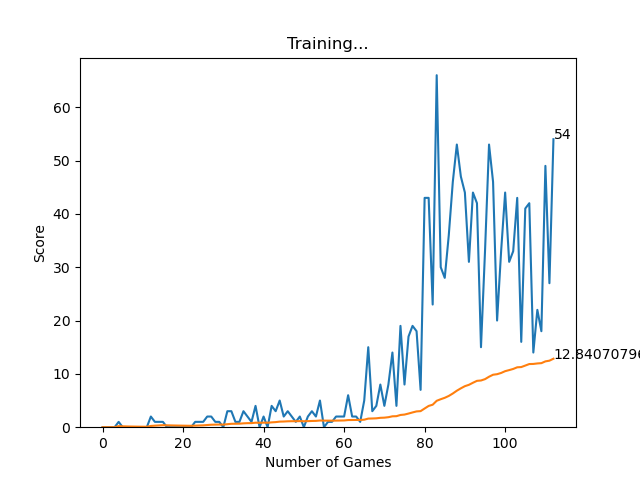Training Progress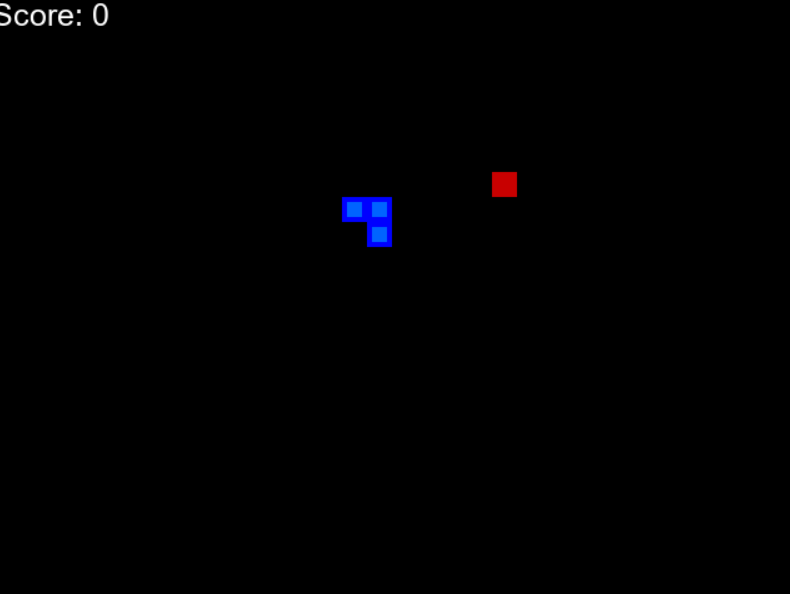Initial TrainingAfter 100 Epochs

Source Code: https://github.com/vedantgoswami/SnakeGameAI

Application:

The Goal of this project is to give an idea that how Reinforcement learning can be applied and how it can be used in Real-world applications such as self-driving cars (eg: AWS DeepRacer), training robots in the assembly line and many more…

Tips:

• Use a separate environment and install all the required modules. (You can use anaconda environment)
• For training the model you can use GPU for faster training.

My Personal Notes arrow_drop_up